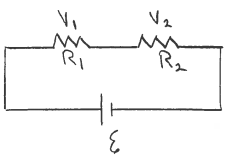# Problem: The circuit shown in the sketch consist of two resistors and a battery with emf  E = 24.0 V and negligible internal resistance, R1 = 2.00 Ω and R2 = 4.00 Ω. What is the voltage across each resistor? V1 =  V2 =

###### FREE Expert Solution
86% (164 ratings)
###### Problem Details

The circuit shown in the sketch consist of two resistors and a battery with emf  E = 24.0 V and negligible internal resistance, R1 = 2.00 Ω and R2 = 4.00 Ω. What is the voltage across each resistor?

V1

V2